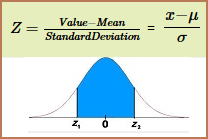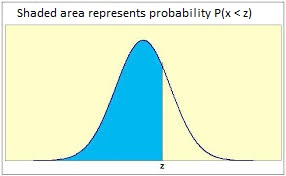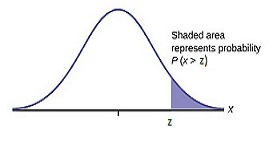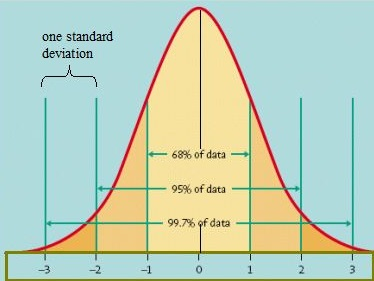#### NORMAL DISTRIBUTION EXAMPLES AND CALCULATOR

A Normal Distribution, sometimes is called the bell curve.

Transfer from Z to Percentile for Normal Distribution

Input Z:

P(%) Left Tailed..:
P(%) Right Tailed:
P(%) Both Tailed.:
Confidence Level.:

Transfer from Percentile to Z for Normal Distribution

Input P (%):

Z score One tailed: Z score Two tailed:

A Normal Distribution

is a distribution that occurs naturally in many situations. For example, the bell curve is seen in tests like the SAT and GRE. The bulk of students will score the average (C), while smaller numbers of students will score a B or D. An even smaller percentage of students score a F or an A. This creates a distribution that resembles a bell (hence the nickname). The bell curve is symmetrical. Half of the data will fall to the left of the mean; half will fall to the right. Many groups follow this type of pattern such as Heights of people, measurement errors, blood pressure, points on a test, IQ scores, salaries... That’s why it’s widely used in business, statistics and in government.

SIX PROBLEM TYPE EXAMPLES (set one):

1. The average score of a statistics test for a class is 85 and standard deviation is 10. Find the probability of a random score falling between 75 and 95.Instruction: Step 1: Use the formula on the above photo to computer z1 and z2

Step 2: Use the z table to find probabilities p1 and p2

Step 3: Find the difference (p2 - p1)*100%

2. The average speed of a car is 65 kmph with a standard deviation of 4. Find the probability that the speed is less than 60 kmph.Instruction: Step 1: Use the formula on the first photo to computer z

Step 2: Use the z table to find probabilities p*100%

3. If mean of a given data for a random value is 81.1 and standard deviation is 4.7, then find the probability of getting a value more than 83.Instruction: Step 1: Use the formula on the first photo to computer z

Step 2: Use the z table to find probabilities p

Step 3: The final answer is: (100% - p*100%)

4. The bottom of 30% of students failed an end of semester exam. The mean for the test is 120 and the standard deviation is 17. What was the passing score?

Instruction: Step 1: Change 30% to decimal

Step 2: Use the z table to find z score with negative sign

Step 3: Compute x = (-z*sigma + muy)

5. The top 30% of test takers pass a particular test. What is the lower cut off for the test if the mean is 72 and the standard deviation is 14?

Instruction: Step 1: Change 30% to decimal

Step 2: Use the z table to find z score with positive sign

Step 3: Compute x = (z*sigma + muy)

6. Researchers want to select people in the middle 60% of the population based on their blood pressure. If the mean blood pressure is 120 and the standard deviation is 8, find the upper and lower readings that would qualify.

Instruction: Step 1: Change 60% to decimal and divide it by 2

Step 2: Compute (0.5 - answer from step 1)

Step 3: Use z table to find z score

Step 4: Compute x1 and x2 by using this formula x = (z*sigma + muy) for positive and negative z

SIX PROBLEM TYPE EXAMPLES (set two):

1. A wild pack of Chihuahuas terrorizing the countryside has a mean height of 7.5 inches, with a standard deviation of 1.5 inches. We feel sorry for the person who had to measure that. What proportion of these Chihuahuas are between 6 and 9 inches tall?

2. Some normal distribution has a mean of 0 and a standard deviation of 1. What is the probability of getting a result less than 1.49?

3. We got a score of 85 on our latest underwater basket-weaving test. If the test scores were normally distributed, with a mean of 78 and a standard deviation of 5, what proportion of the class would likely score better than we did?

4. Scores on an English test are normally distributed with a mean of 37.3 and a standard deviation of 8. Find the score that separates the bottom 41%?

5. Suppose that replacement times for washing machines are normally distributed with a mean of 8.4 years and a standard deviation of 2 years. Find the replacement time that separates the top 18% times.

6. The weights of certain machine components are normally distributed with a mean of 8.01 g and a standard deviation of 0.06 g. Find the two weights that are the boundaries for the middle 90%?

FIND Z and PROBABILITY for NORMAL DISTRIBUTIONEnter the mean (average) and the standard deviation first.

Input Mean:
Stand. Dev:

THEN CHOOSE ONE OF THE FOLLOWING SIX BASIC PROBLEM TYPES (on the left)

TYPE ONE: (Problems with keyword "between") Enter X-min and X-max

and

Prob. (%):
Z score 1:
Z score 2:

TYPE TWO: (Problems with keyword "less than") Enter X

Probability (%): Z score:

TYPE THREE: (Problems with keyword "more than") Enter X

Probability (%): Z score:

TYPE FOUR: (Problems with Lower Cut Off) Enter lower cut off %:

Z score: X value:

TYPE FIVE: (Problems with Upper Cut Off) Enter upper cut off %:

Z score: X value:

TYPE SIX: (Problems with Middle Percent) Enter middle percent

Z score:
X1 value: X2 value: